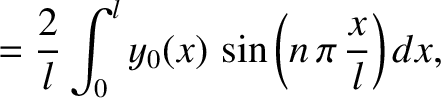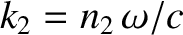Next: Cylindrical Waves Up: Multi-Dimensional Waves Previous: Plane Waves

# Three-Dimensional Wave Equation

We have already seen that the one-dimensional plane wave solution, (529), satisfies the one-dimensional wave equation,(536)

whereis the characteristic wave speed of the medium through which the wave propagates. (See Section 7.2.) Likewise, the three-dimensional plane wave solution, (533), satisfies the three-dimensional wave equation (see Exercise 1),(537)

Richard Fitzpatrick 2013-04-08